# 多头注意力2022年10月16日07:41:51

4706字阅读15分41秒

## 简介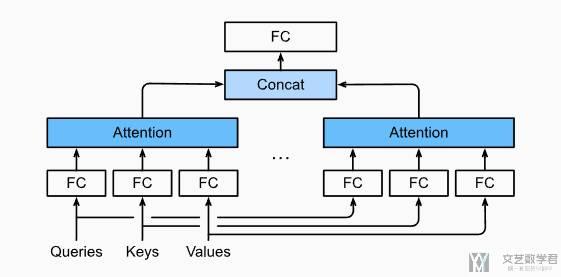## 多头注意力模型

### 模型描述--图解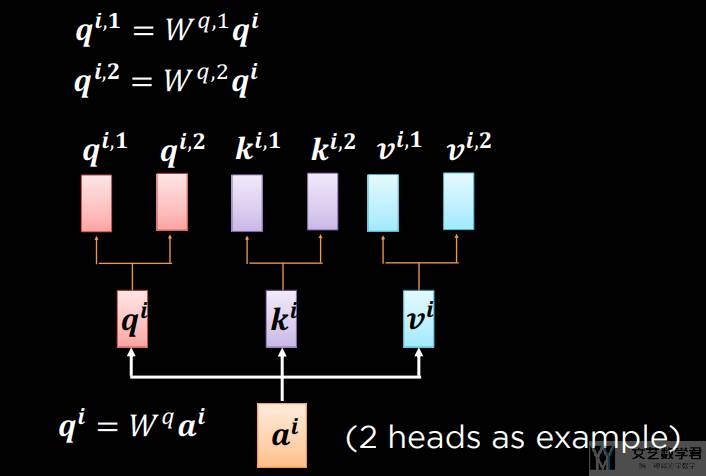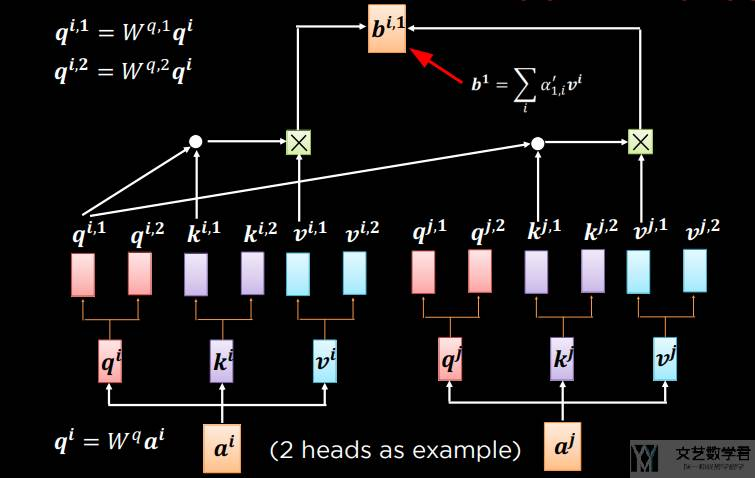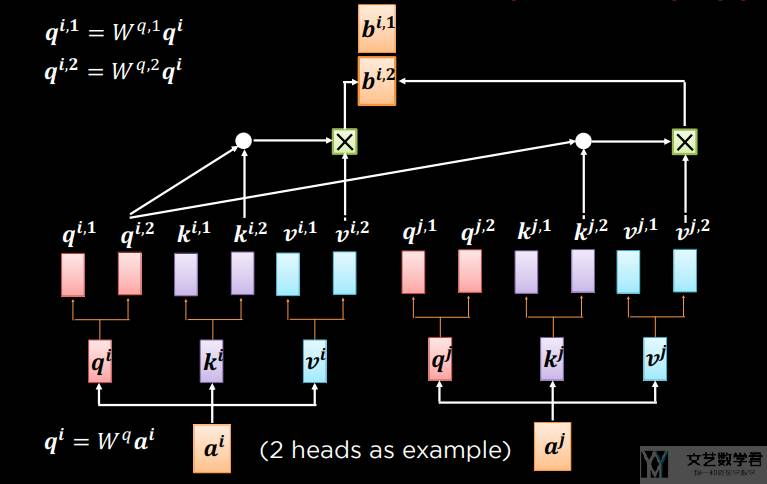### 模型解释-数学语言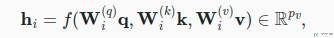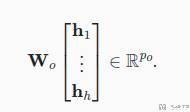## 多头注意力代码实现

1. batch_size = 2 # batch 数量
3. num_queries = 4 # query 的数量为 4
4. num_kvpairs =  6 # kvpair 的数量为 6
5. valid_lens = torch.tensor([3, 2])
6. X = torch.ones((batch_size, num_queries, 10)) # query
7. Y = torch.ones((batch_size, num_kvpairs, 20)) # key, value
8. print(X.shape, Y.shape)
9. # torch.Size([2, 4, 10]) torch.Size([2, 6, 20])

3.     """
4.     # 输入 X 的形状:(batch_size，查询或者“键－值”对的个数，num_hiddens)
6.     # 有多少个 head, 做一下切分, 比如会从 torch.Size([2, 4, 100]) --> torch.Size([2, 4, 5, 20])
7.     X = X.reshape(X.shape, X.shape, num_heads, -1)
9.     # 交换维度, 将 num_heads 和 查询或者“键－值”对的个数 交换
10.     # 此时的大小为 torch.Size([2, 5, 4, 20])
11.     X = X.permute(0, 2, 1, 3)
13.     # 最终大小变为 torch.Size([10, 4, 20])
14.     return X.reshape(-1, X.shape, X.shape)

1. num_hiddens = 100
2. W_q = nn.LazyLinear(num_hiddens, bias=False)
3. W_k = nn.LazyLinear(num_hiddens, bias=False)
4. W_v = nn.LazyLinear(num_hiddens, bias=False)
8. print(queries.shape, keys.shape, values.shape)
9. # torch.Size([10, 4, 20]) torch.Size([10, 6, 20]) torch.Size([10, 6, 20])

1. attention = DotProductAttention(0.1)
2. valid_lens = torch.repeat_interleave(valid_lens, repeats=num_heads, dim=0)
3. output = attention(queries, keysvalues, valid_lens)
4. print(output.shape) # 计算每个 query 的值, 为 (10, 4, 20)
5. # torch.Size([10, 4, 20])

2.     """逆转transpose_qkv函数的操作
3.     """
4.     # 将 num_heads 的维度信息从 batch size 中拿出来
5.     # 也就是 torch.Size([10, 4, 20]) --> torch.Size([2, 5, 4, 20]), 这里 num_head = 5
6.     X = X.reshape(-1, num_heads, X.shape, X.shape)
7.     # 交换 num_heads 与 查询或者“键－值”对的个数
8.     # 此时从 torch.Size([2, 5, 4, 20]) --> torch.Size([2, 4, 5, 20])
9.     X = X.permute(0, 2, 1, 3)
11.     # 从 torch.Size([2, 4, 5, 20]) --> torch.Size([2, 4, 100])
12.     return X.reshape(X.shape, X.shape, -1)

2. # 将 num_head 从 batch_size 中拿出来, torch.Size([10, 4, 20]) --> torch.Size([2, 4, 100])
3. print(output_concat.shape)
4. # 这里的 100 相当于是 multi-head 集和后的结果1. W_o = nn.LazyLinear(32, bias=False)
2. result = W_o(output_concat)
3. print(result.shape) # torch.Size([2, 4, 100]) --> torch.Size([2, 4, 32])
4. # torch.Size([2, 4, 32])

• 微信公众号
• 关注微信公众号
•• QQ群
• 我们的QQ群号
•• 本文由 发表于 2022年10月16日07:41:51
• 转载请务必保留本文链接：https://mathpretty.com/15210.html